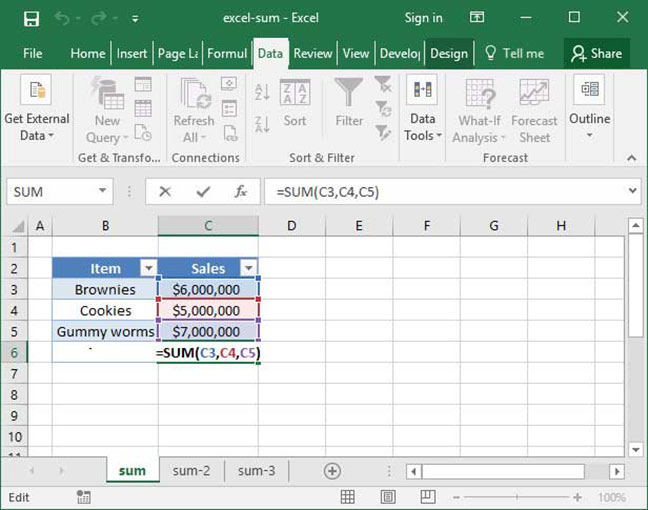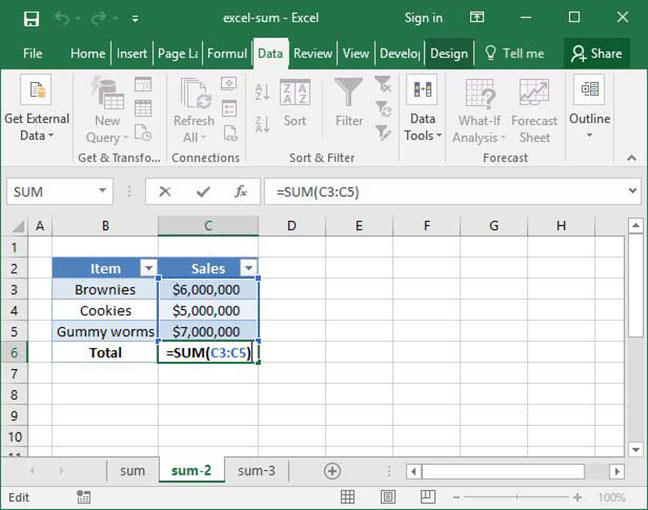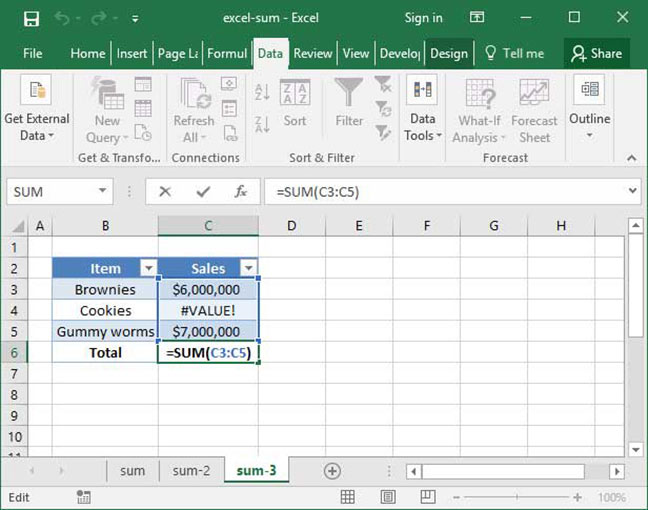# Excel's SUM function

Let's start our overview of mathematical functions with Excel's most basic, and commonly-used, tool: SUM.

The SUM function does just what it sounds like: It sums up each of its arguments and outputs the total. Let's take a look at how this simple function works.

## Using SUM

The basic syntax for the SUM function is as follows:

`=SUM(number_1, number_2...)`

Note that the SUM function will take as many number arguments as you want — you can sum up any number of values to get your total! Let's take a look at a couple examples of SUM used with simple numbers as its arguments:

`=SUM(3, 7)Output: 10`
`=SUM(15, 22)Output: 37`
`=SUM(15, 22,13, 1)Output: 51`

Like all functions, SUM can also be used with cell references as its arguments. Take, for example, the following sheet, in which we SUM up the sales values for a number of SnackWorld product categories:`=SUM(C3, C4, C5)Output: \$18,000,000`

The above formula outputs \$18,000,000, because the sum of \$6,000,000, \$5,000,000, and \$7,000,000 is \$18,000,000.

## SUM with a range of cells

As we've seen above, SUM works great with single cells. But it also offers a shortcut for data input when working with large ranges of cells: you can input an entire cell range rather than a single cell reference, and Excel will automatically sum up everything in that range.

Take a look at the below spreadsheet, in which we've rewritten the formula above with a more simple version that uses SUM based on a range rather than individual cells:`=SUM(C3:C5)Output: \$18,000,000`

This formula also outputs \$18,000,000, because it performs the SUM of all the cells in the range C3:C5. This is a helpful shortcut when you want to SUM large quantities of data that appear over many cells.

## Errors in your SUM formula

It's important to note that the SUM formula will return an error if any of the values that you attempt to add up contain errors. The following example shows what happens when one of the values we try to SUM contains the #VALUE! error:`=SUM(C3:C5)Output: #VALUE!`

As you can see, SUM isn't working in this situation. If you're getting errors like #VALUE! or #DIV/0! as the output of your SUM, check to ensure that each value you're adding up is a number and not an error.